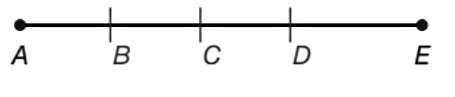Chapter 1.2, Problem 45E### Elementary Geometry for College St...

6th Edition
Daniel C. Alexander + 1 other
ISBN: 9781285195698

#### Solutions

Chapter
Section### Elementary Geometry for College St...

6th Edition
Daniel C. Alexander + 1 other
ISBN: 9781285195698
Textbook Problem
1 views

# A compass was used to mark off three congruent segment, A B ¯ , B C ¯ , and C D ¯ . Thus, A D ¯ has been trisected at points B and C . If A D = 32.7 , how long is A B ¯ ?To determine

To find:

The length of AB¯.

Explanation

Calculation:

Given,

AB¯, BC¯, and CD¯ are congraent.

Since, the line segment AD¯ is trisected, the length of AD¯ divided by 3 gives the length of measure of each of the segments

### Still sussing out bartleby?

Check out a sample textbook solution.

See a sample solution

#### The Solution to Your Study Problems

Bartleby provides explanations to thousands of textbook problems written by our experts, many with advanced degrees!

Get Started

#### Find more solutions based on key concepts